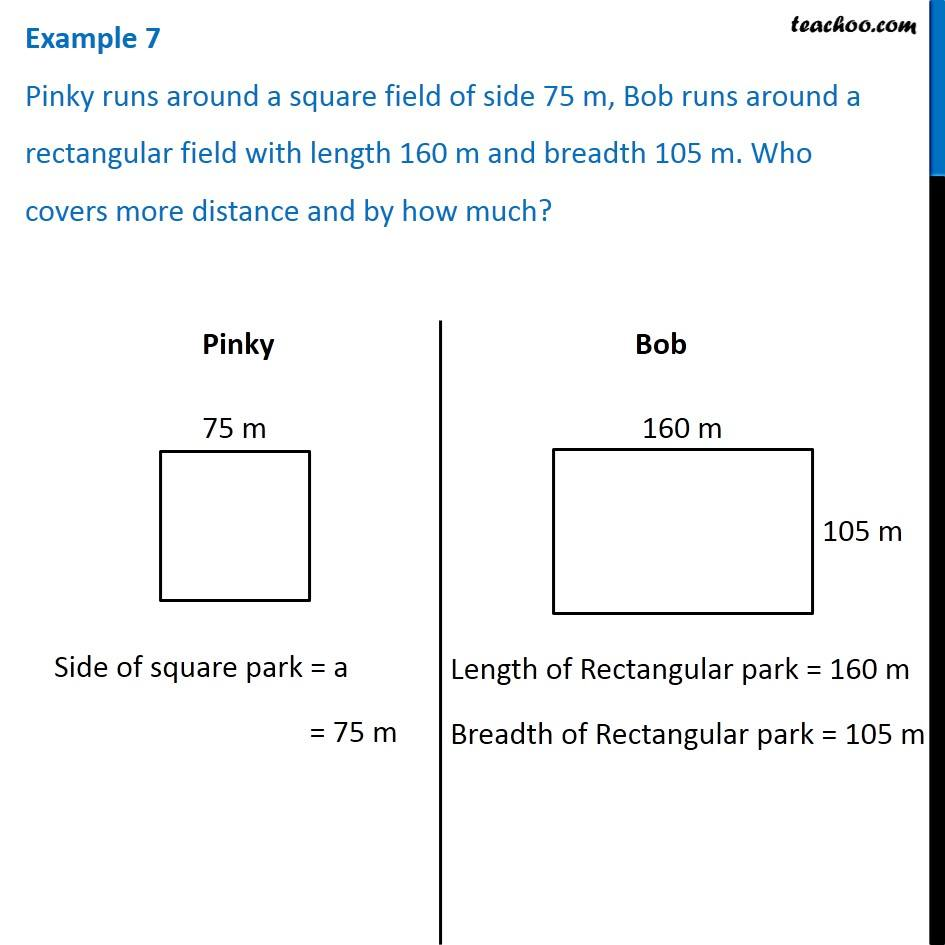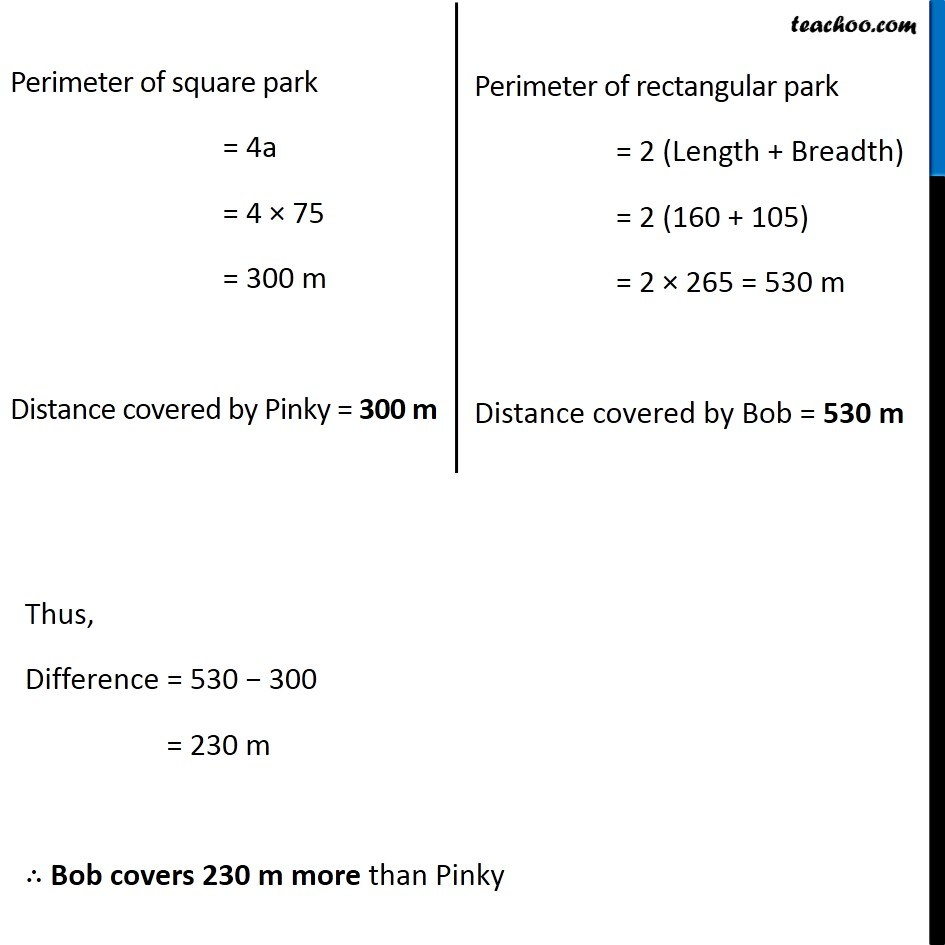Perimeter of Square

Chapter 10 Class 6 Mensuration
Concept wiseLearn in your speed, with individual attention - Teachoo Maths 1-on-1 Class

### Transcript

Example 7 Pinky runs around a square field of side 75 m, Bob runs around a rectangular field with length 160 m and breadth 105 m. Who covers more distance and by how much? Side of square park = a = 75 m Length of Rectangular park = 160 m Breadth of Rectangular park = 105 m Perimeter of square park = 4a = 4 × 75 = 300 m Distance covered by Pinky = 300 m Perimeter of rectangular park = 2 (Length + Breadth) = 2 (160 + 105) = 2 × 265 = 530 m Distance covered by Bob = 530 m Thus, Difference = 530 − 300 = 230 m ∴ Bob covers 230 m more than Pinky